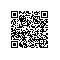# HTML5标签的语义认知和理解（2）

1. <article>
2.     <hgroup>
3.         <h1>
4.             主标题
5.         </h1>
6.         <h2>
7.             副标题
8.         </h2>
9.     </hgroup>
10.     <section>
11.         <h1>
12.             第一章</h1>
13.         <p>
14.             自然段</p>
15.         <p>
16.             自然段</p>
17.         <p>
18.             自然段</p>
19.         <p>
20.             自然段</p>
21.     </section>
22.     <section>
23.         <h1>
24.             第二章</h1>
25.         <p>
26.             自然段</p>
27.         <p>
28.             自然段</p>
29.         <p>
30.             自然段</p>
31.         <p>
32.             自然段</p>
33.     </section>
34.     <footer>
35.         <time>发表日期</time>
36.     </footer>
37. </article>

1. <aside>
2.     <article>
4.             <h1>
5.                 向你推荐</h1>
6.             <h2>
7.                 喜欢这篇文章的人还喜欢……</h2>
9.         <ol>
10.             <li>
11.                 <section>
12.                     <article>
13.                         <h1>
14.                             文章标题</h1>
15.                         <section>
16.                             <p>
17.                                 摘要</p>
18.                         </section>
19.                     </article>
20.                 </section>
21.             </li>
22.             <li>
23.                 <section>
24.                     <article>
25.                         <h1>
26.                             文章标题</h1>
27.                         <section>
28.                             <p>
29.                                 摘要</p>
30.                         </section>
31.                     </article>
32.                 </section>
33.             </li>
34.         </ol>
35.         <footer>
36.             <article>
37.                 <h1>
38.                     其他更多推荐</h1>
39.                 <ol>
40.                     <li>推荐1</li>
41.                     <li>推荐2</li>
42.                     <li>推荐3</li>
43.                 </ol>
44.             </article>
45.         </footer>
46.     </article>
47. </aside>

1. <figure>
2.     <img alt="" />
3.     <img alt="" />
4.     <img alt="" />
5.     <img alt="" />
6.     <figcaption>这四个产品是我们最新的……</figcaption>
7. </figure>使用钉钉扫一扫加入圈子
+ 订阅